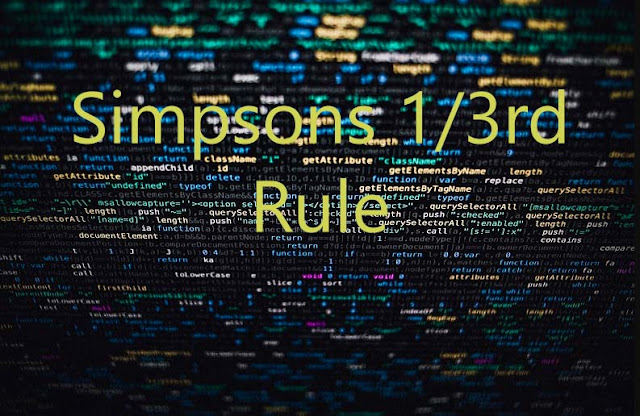# Fortran ~ Simpson's 1/3rd Rule - Ishwaranand

## Fortran ~ Simpson's 1/3rd Rule

### By putting n=2 into a basic equation by integration and taking the curve through (X0, Y0), (X1, Y1) and (X2, Y2) as a polynomial of degree 2 so that difference of order Three and greater than three become zero, than we get.F(X)=3.12*X**3+1.54*X**2-6.23This is known as Simpson's 1/3rd Rule.Image Source ~ Crafted With ©Ishwaranand - 2020 ~ Image by ©IshwaranandRemarks Simpson's 1/3rd RuleSimpson's 1/3rd rule requires the division of the interval [X0, Xn] into an even number od sub-intervals of width h.In this rule, the interpolating polynomial is of degree 2.Therefore this rule is also known as a parabolic rule. (adsbygoogle = window.adsbygoogle || []).push({});

PROGRAM SIMPSON
C
C         PROGRAM TO DEMONSTRATE APPLICATION OF SIMPSONS 1/3rd RULE
C
C        THIS PROGRAM NUMERICALLY INTEGRATES GIVEN EXPRESSION WITHIN
C        SPECIFIED LIMITS OF INTEGRATION
C
F(X)=3.12*X**3+1.54*X**2-6.23
C
WRITE (*,*)'LIMITS OF INTEGRATION (A,B)'
WRITE(*,*)'NUMBER OF STRIPES(Number must be Even)'
IF(NS/2*2.NE.NS)THEN
WRITE(*,*)'NUMBER OF STRIPES (must be Even)'
WRITE(*,*)'PROGRAM TERMINATED...'
STOP
ENDIF
C         STRIP SIZE
H=(B-A)/NS
AREA=F(A)+F(B)
DO 100 X=A+H,B-H,2*H
AREA=AREA+4.0*F(X)
100      CONTINUE
DO 200 X=A+2*H,B-2*H,2*H
AREA=AREA+2.0*F(X)
200      CONTINUE
AREA=AREA*H/3.0
WRITE(*,*)'AREA UNDER THE CURVE=',AREA
STOP
END

#OUTPUT

LIMIT OF INTEGRATION (A, B)
1 2
NUMBER OF STRIPS (Number must be Even)
2
AREA UNDER THE CURVE = 54.546665